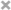### 我国传统的长度单位

```1里=150丈=500米
2里=1公里(1000米)
1丈=10尺
1丈=3.33米
1尺=3.33分米```

### 英制长度单位

```英里(mile)1英里=1760码=5280英尺=1.609344公里
码(yard,yd) 1码=3英尺=0.9144米
英寻(f,fath,Fa,ftm) 1英寻=2码=1.8288米
浪(furlong) 1浪=220码=201.17米
英尺(foot,ft,复数为feet) 1英尺=12英寸=30.48厘米
英寸(inch,in) 1英寸=2.54厘米```

### 天文学长度单位

```1光年=9.4653×10^12km
1秒差距=3.2616光年
1天文单位≈1.496亿千米```

### 其他的长度单位还有

```1PM(拍米)=1×10^15m
1Gm(京米/吉米)=1×10^9m
1Mm(兆米)=1×10^6m
1km(千米)=1×10^3m
1dm(分米)=1×10^(-1)m
1cm(厘米)=1×10^(-2)m
1mm(毫米)=1×10^(-3)m
1dmm(丝米)=1×10^(-4)m
1cmm(忽米)=1×10^(-5)m
1μm(微米)=1×10^(-6)m
1nm(纳米)=1×10^(-9)m
1pm(皮米)=1×10^(-12)m
1fm(飞米)=1×10^(-15)m
1am(阿米)=1×10^(-18)m```